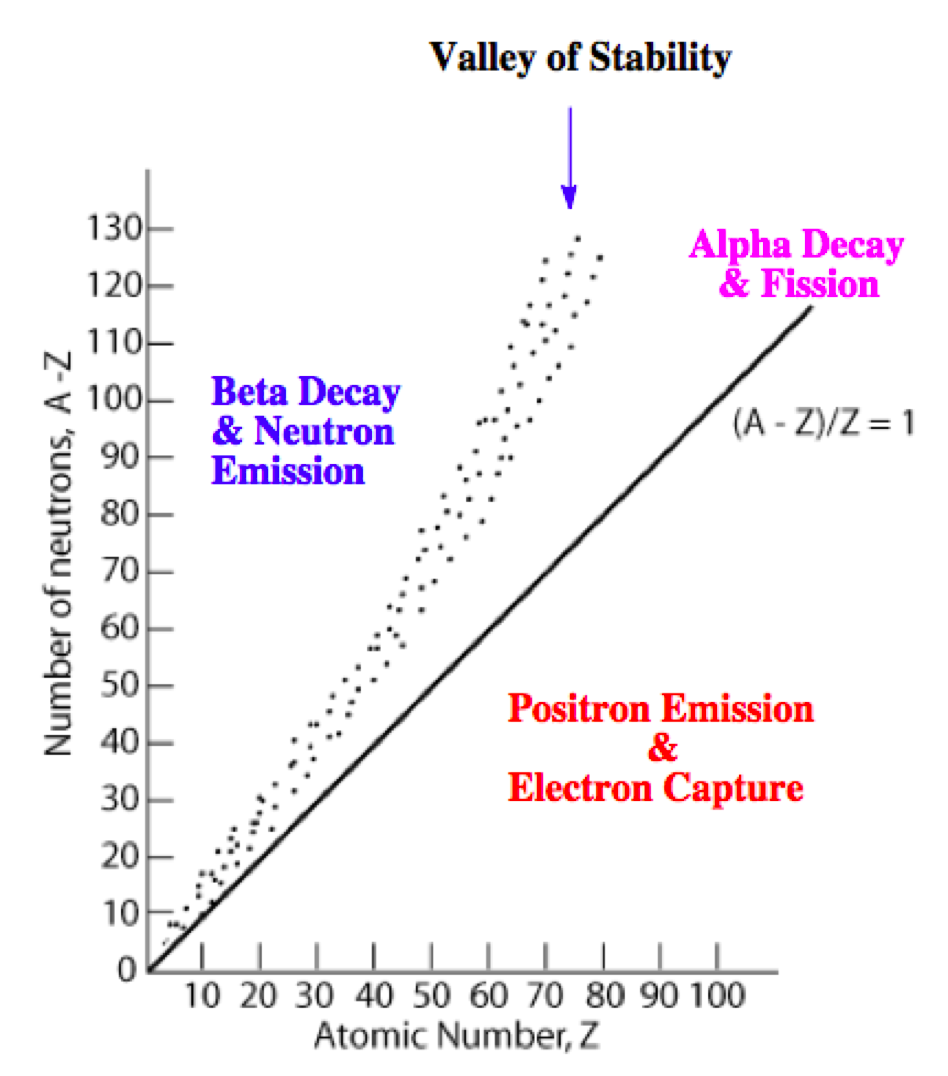# Problem: Chlorine has two stable nuclides, 35Cl and 37Cl. In contrast, 36Cl is a radioactive nuclide that decays by beta emission.Based on the empirical rules about nuclear stability, explain why the nucleus of 36Cl is less stable than either 35Cl or 37Cl.

###### FREE Expert Solution

We’re being asked to explain why the nucleus of 36Cl is less stable than either 35Cl or 37Cl

Recall that the stability of an isotope depends on the ratio of neutrons to protons (N/Z). This means for stable isotopes with:

• Z ≤ 20; the N/Z ratio should be equal to 1.0

• 20 < Z ≤ 40; the N/Z ratio should be equal to 1.25

• 40 < Z ≤ 80; the N/Z ratio should be equal to 1.50

• Z < 83; the stable nuclide does not exist

The N/Z values for stable isotopes form the valley or band of stability:This means for isotopes:

• above the valley of stability: there too many neutrons in the isotope

Mode of decay: Either beta decay (0–1β) or neutron emission (10n; not in the choices)

• below the valley of stability: there too many protons in the isotope

Mode of decay: Either positron emission (01e) or electron capture (0–1e)

• with atomic mass ≥ 200 amu: there too many protons and neutrons in the isotope

Mode of decay: alpha decay (42α)###### Problem Details

Chlorine has two stable nuclides, 35Cl and 37Cl. In contrast, 36Cl is a radioactive nuclide that decays by beta emission.

Based on the empirical rules about nuclear stability, explain why the nucleus of 36Cl is less stable than either 35Cl or 37Cl.